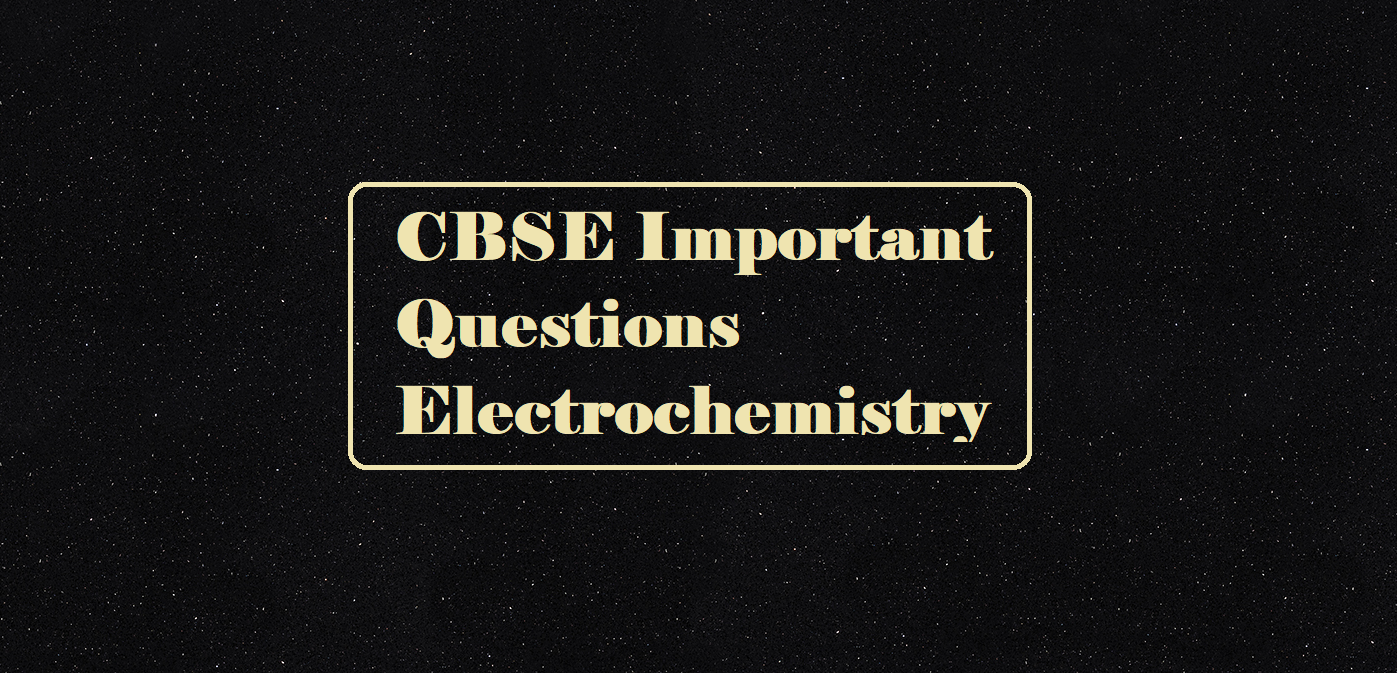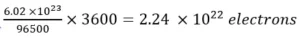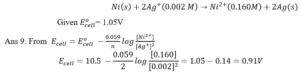# CBSE Important Questions Electrochemistry# CBSE Important Questions Electrochemistry

CBSE Important Questions Electrochemistry

Que 1. Why conductivity of a solution does decrease with dilution?
Ans 1. On dilution, the number of ions per unit volume decreases thus conductivity decreases.

Que 2. If 0.5 ampere current flows through a wire for 2 hours. Calculate the number of electrons through the wire.
Ans2. Q = I × t = 0.5 × 2 × 60 × 60 = 3600 C
96500 C is equivalent to charge on 1 mole = 6.022 × 1023 electrons
3600 C is equivalent to charge on  =Que 3. How much charge is required in the reduction of one mole of Al3+ to Al?
Ans 3. The reaction is Al3+ + 3 e– → Al
... Quantity of charge required = 3F = 3 × 96500= 289500 C

Que 4. Write the importance of salt bridge in a Galvanic cell?
Ans 4. i) To complete the inner circuit &
ii) To maintain electrical neutrality.

Que 5. What is meant by cell constant?
Ans 5.  It is the ratio of the distance between electrodes & area of cross-section.

Que 6. Why an aqueous NaCl on electrolysis gives H2 gas at the cathode & not sodium?
Ans 6. The standard reduction potential of water is greater than that of sodium.

Que 7. How much electricity (Faraday) is required to produce 20 g of Ca from Calcium Chloride?
Ans 7. According to the statement, the reaction involved is
Ca2+ + 2 e– → Ca
For 1 mole Ca (40 g Ca require) = 2F
20 gm i.e 0.5 mole Ca require electricity = 1 F

Que 8. Why does a Galvanic cell become dead after some time?
Ans 8. As the reaction in the cell precedes the concentration of ions in one half cell increases while in the other half cell decreases hence electrode potential also changes. When concentration becomes equal the E.M.F becomes zero.

Que 9. Calculate the E.M.F of the following cell:Que 10. What is electrochemical series? Explain its importance.
Ans 10. Electrochemical series: the arrangement of metals & non-metals in increasing order of their standard electrode potentials is called electrochemical series.
With the help of electrochemical series, we can find standard electrode potentials of various species, and using these values we can determine the pH of solutions, solubility product, equilibrium constant, predict the feasibility of a reaction.